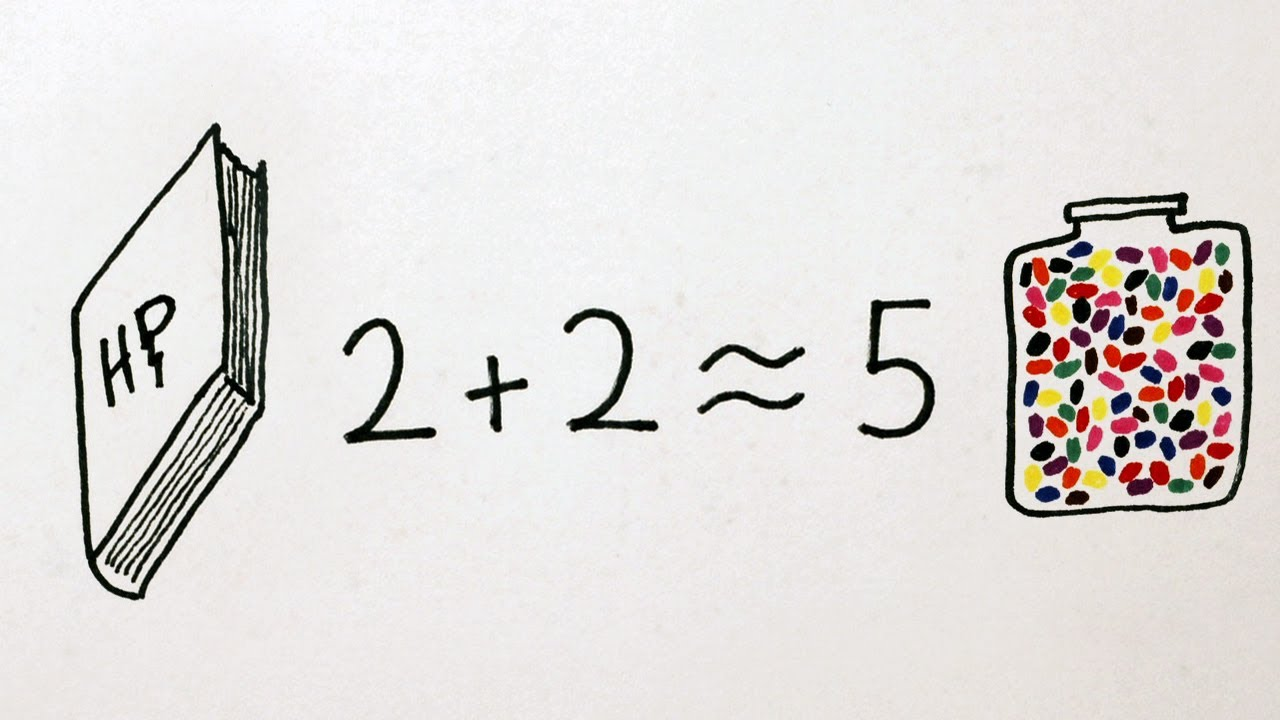# How To Weigh A Million Dollar Bills Without A ScaleIn physics, approximations are important. Developing a predictive model for how something will move/behave/react based on information from another object or scenario can provide a helpful frame of reference. To illustrate this point, the always excellent YouTube series MinutePhysics shows us how to weigh a million US dollar bills without having a scale, a single bill, or the knowledge of how much a dollar weighs.

Assuming a physicist can use their knowledge of other materials or objects to estimate the dimensions, weight and density of an object with a reasonable degree of accuracy, they can use proportional maths to convert what they know into what they don’t know (in this case the video uses the volume of a book and the density of water to approximate the weight of a dollar bill). And, as the video shows, their approximation was pretty damn close. [MinutePhysics]